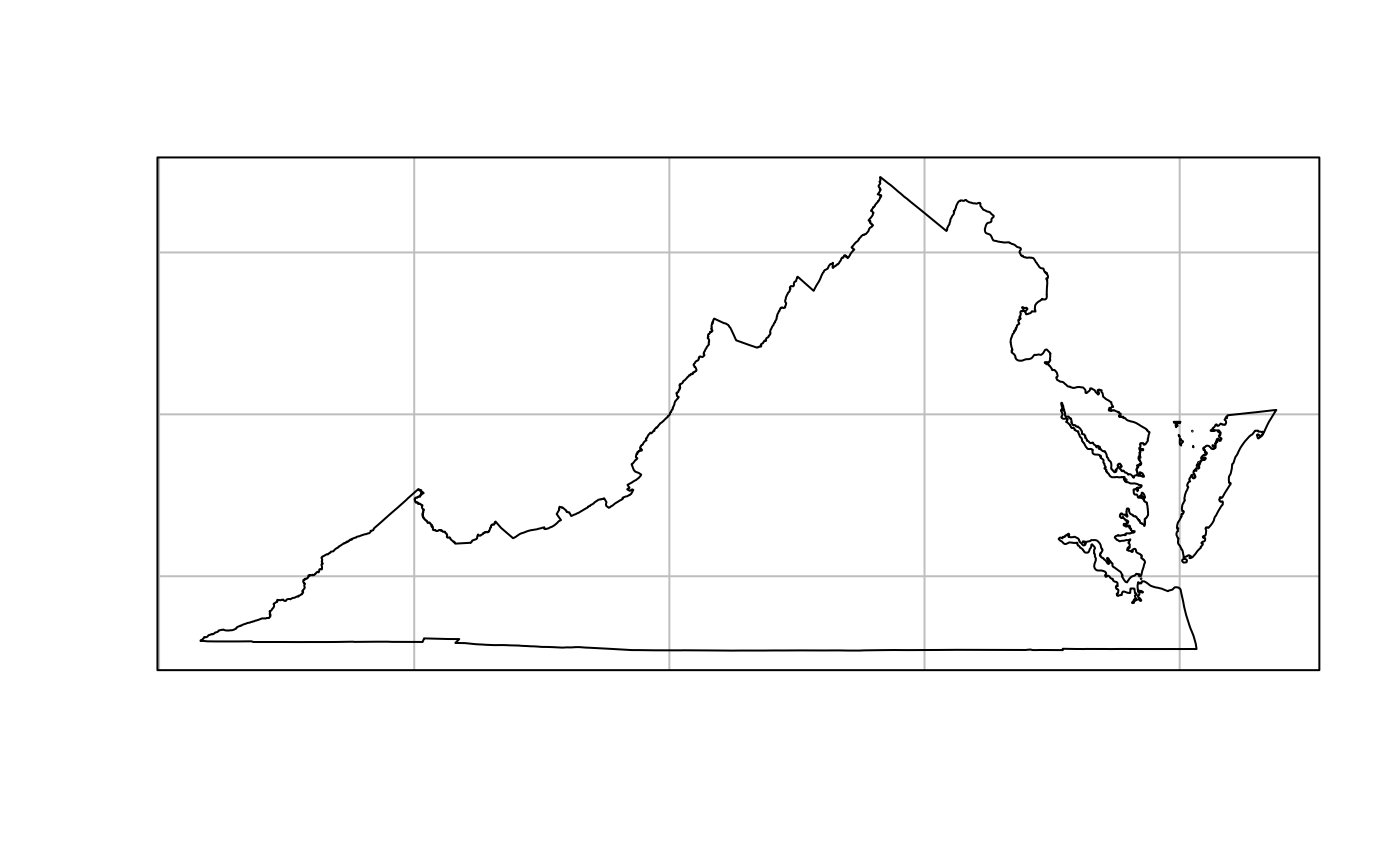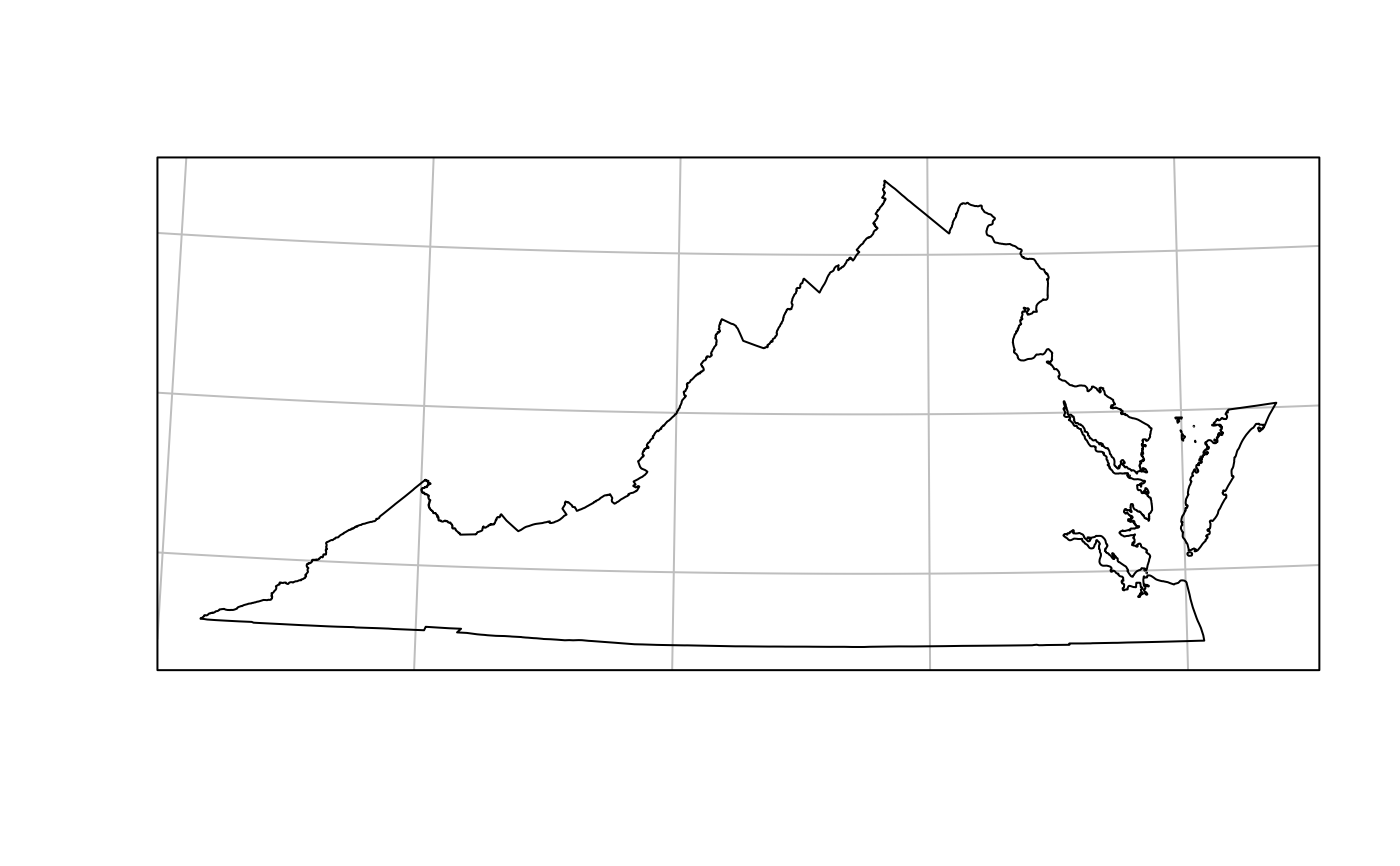Get EPSG codes or PROJ.4 codes for projections from the State Plane Coordinate System.

## Usage

``state_plane(state, plane_id = NULL, type = c("epsg", "proj4"))``

## Arguments

state

The postal code for the state.

plane_id

The state plane geographic zone identifier. A `NULL` value will return a projection for the entire state.

type

The type of output to return: either an EPSG code or PROJ4 string.

## Value

Either a PROJ4 string as a character vector or an EPSG code as an integer.

For documentation of the underlying State Plane Coordinate System projection data frame, see `state_proj`.

## Examples

``````if (require(USAboundariesData)) {
state_plane(state = "MA", type = "epsg")
state_plane(state = "MA", type = "proj4")
state_plane(state = "MA", plane_id = "island", type = "epsg")
state_plane(state = "MA", plane_id = "island", type = "proj4")

# Show the difference made by a state plane projection
if (require(sf)) {
va <- us_states(states = "VA", resolution = "high")
plot(st_geometry(va), graticule = TRUE)
va <- st_transform(va, state_plane("VA"))
plot(st_geometry(va), graticule = TRUE)
}
}